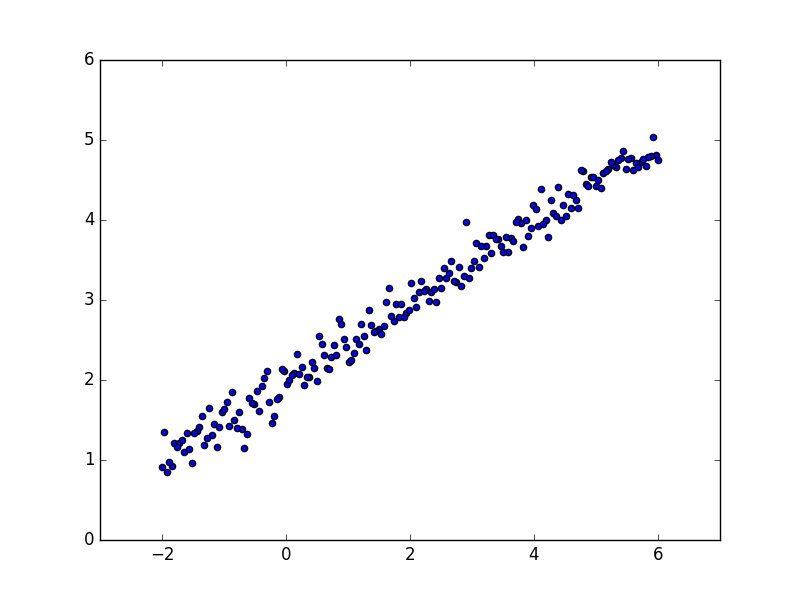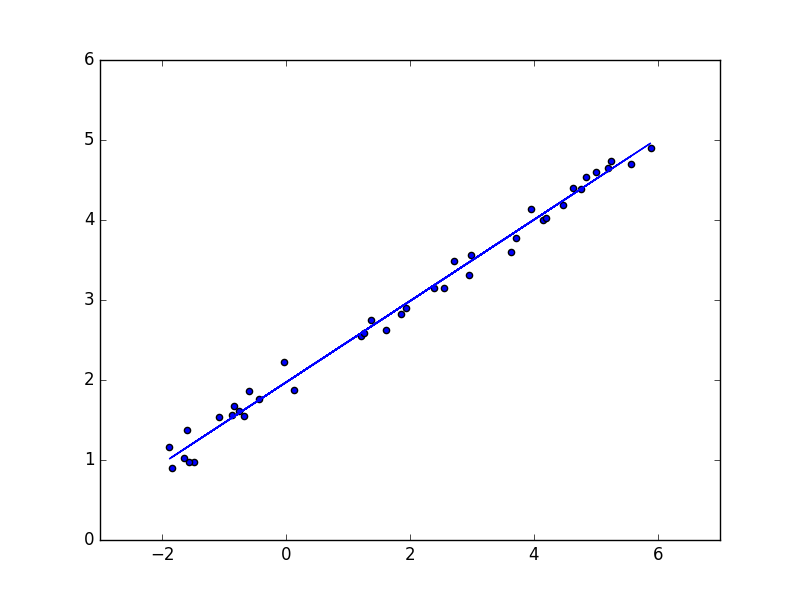Keras实例教程(1)

Model definition → Model compilation → Training → Evaluation and Prediction

import numpy as np
import matplotlib.pyplot as plt

X = np.linspace(-2, 6, 200)
np.random.shuffle(X)
Y = 0.5 * X + 2 + 0.15 * np.random.randn(200,)

# plot data
plt.scatter(X, Y)
plt.show()

X_train, Y_train = X[:160], Y[:160]     # train first 160 data points
X_test, Y_test = X[160:], Y[160:]       # test remaining 40 data pointsfrom keras.models import Sequential
from keras.layers import Dense

model = Sequential()
model.add(Dense(output_dim = 1, input_dim = 1))

model.compile(loss='mse', optimizer='sgd')

from keras.optimizers import SGD
model.compile(loss='mse', optimizer=SGD(lr=0.01, momentum=0.9, nesterov=True))

model.fit(x_train, y_train, epochs=100, batch_size=64)

model.train_on_batch(x_batch, y_batch)

print('Training -----------')
for step in range(100):
cost = model.train_on_batch(X_train, Y_train)
if step % 20 == 0:
print('train cost: ', cost)

Training -----------
train cost:  0.0290748
train cost:  0.0276375
train cost:  0.026696
train cost:  0.0260792
train cost:  0.0256752

cost = model.evaluate(X_test, Y_test, batch_size=40)

print('\nTesting ------------')
loss_and_metrics = model.evaluate(X_test, Y_test, batch_size=40)
print('test cost:', cost)
W, b = model.layers.get_weights()
print('Weights=', W, '\nbiases=', b)

Testing ------------
40/40 [==============================] - 0s
test cost: 0.0254213
Weights= [[ 0.50829154]]
biases= [ 1.9707619]

Y_pred = model.predict(X_test)
plt.scatter(X_test, Y_test)
plt.plot(X_test, Y_pred)
plt.show()